## 一. ROC曲线

### 2、针对一个二分类问题，将实例分成正类(postive)或者负类(negative)。但是实际中分类时，会出现四种情况.

(1)若一个实例是正类并且被预测为正类，即为真正类(True Postive TP)

(2)若一个实例是正类，但是被预测成为负类，即为假负类(False Negative FN)

(3)若一个实例是负类，但是被预测成为正类，即为假正类(False Postive FP)

(4)若一个实例是负类，但是被预测成为负类，即为真负类(True Negative TN)

TP:正确的肯定数目

FN:漏报，没有找到正确匹配的数目

FP:误报，没有的匹配不正确

TN:正确拒绝的非匹配数目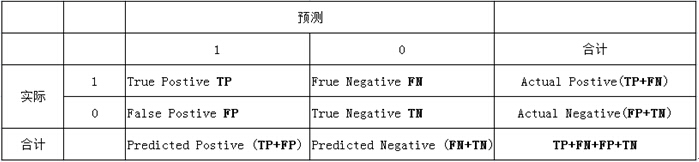(1)真正类率(True Postive Rate)TPR: TP/(TP+FN),代表分类器预测的正类中实际正实例占所有正实例的比例。

(2)负正类率(False Postive Rate)FPR: FP/(FP+TN)，代表分类器预测的正类中实际负实例占所有负实例的比例。

(3)真负类率(True Negative Rate)TNR: TN/(FP+TN),代表分类器预测的负类中实际负实例占所有负实例的比例，TNR=1-FPR。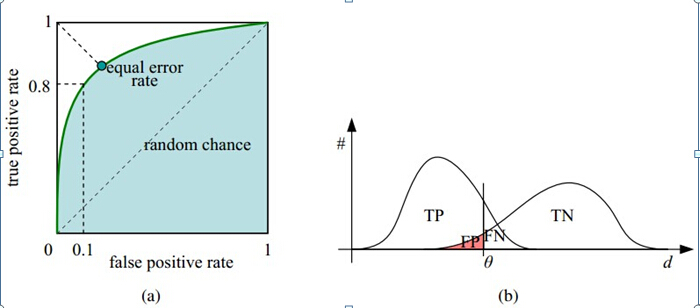## 二. 如何画roc曲线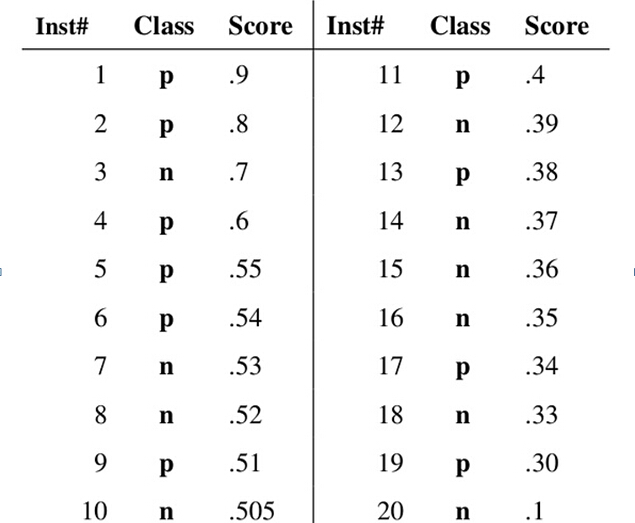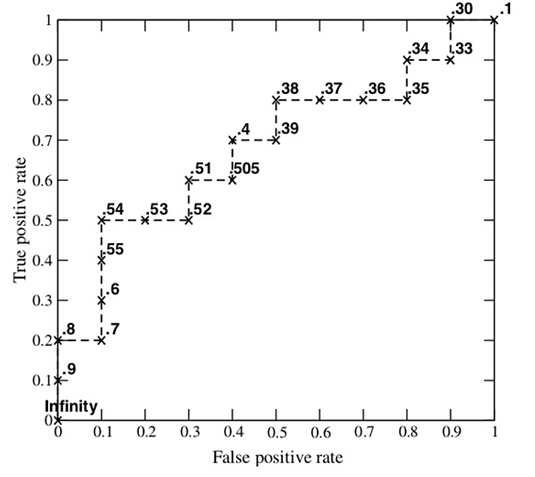### 上图ROC曲线一些点怎么得到的分析

• Score = 0.9时，样本1被认为（预测）是正类（P）。此时根据混淆矩阵有： TP = 1, FN = 9, FP = 0，TN = 10。
这是因为样本1为正类（P）被正确预测为正类（P），因此TP = 1; 其他实际正类类别（class为P的）样本有9个，它们被预测为负类（N）了，因此FN = 9; 样本实际为负类（N）的，被预测为正类（P）的没有一个，因此FP = 0, 其他实际负类类别（class为N的）样本有10个，它们全部被预测为负类（N）了，因此TN = 10 。故TPR = 1/(1+9) = 0.1
• Score = 0.8时， 同理有： TP = 2, FN = 8，FP = 0, TN = 10。 故TPR = 2/(2+8) = 0.2
• Score = 0.7时，有： TP = 2, FN = 8，FP = 1, TN = 9。 故TPR = 2/(2+8) = 0.2
• Score = 0.6时，有：TP = 3, FN = 7，FP = 1， TN = 9。 故TPR = 3/(3+7) = 0.3
以此类推

## 三 为什么使用Roc和Auc评价分类器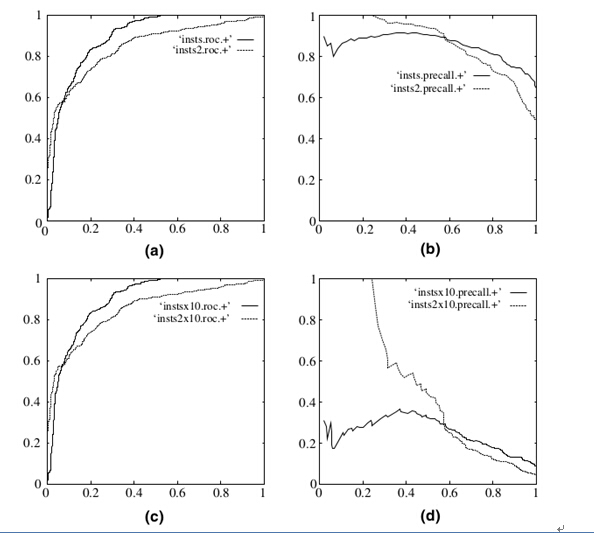(a)和(b)展示的是分类其在原始测试集(正负样本分布平衡)的结果，(c)(d)是将测试集中负样本的数量增加到原来的10倍后，分类器的结果，可以明显的看出，ROC曲线基本保持原貌，而Precision-Recall曲线变化较大。

## 绘制ROC曲线计算AUC值

• case1: 只去前面36行样本数据，txt文件名为：evaluate_result2
'''

'''
#绘制二分类ROC曲线
import matplotlib.pyplot as plt
from math import log, exp, sqrt

evaluate_result = './evaluate_result2.txt'

db  = [ ]   #[score,nonclk,clk]
pos, neg = 0, 0
with open(evaluate_result, 'r') as fs:
for line in fs:
nonclk, clk, score = line.strip().split('\t')  #变成列表list形式
nonclk = int(nonclk)
clk = int(clk)
db.append([score,nonclk,clk])

score = float(score)
neg = neg + nonclk
#print("neg数量====", neg, end = '')
pos = pos + clk
#print(",  pos数量====", pos)

#print("db= ",db)

db = sorted(db, key=lambda x:x, reverse=True)  #对ad的score进行降序排序
#print("db_reverse= ",db)

#计算ROC坐标点
xy_arr = [ ]
tp, fp = 0., 0.

for i in range(len(db)):
fp += db[i]
#print("fp ===", fp, end = '')
tp += db[i]
#print(",   tp ===",tp)
xy_arr.append([fp/neg, tp/pos])   #fp除以negative数目， tp除以positive数目。作为坐标点
#print(xy_arr)  #[[0.06666666666666667, 0.0], [0.06666666666666667, 0.029411764705882353],...]

# 计算曲线下面积
auc = 0.
prev_x = 0
for x, y in xy_arr:   #  x= fp/neg， y = tp/pos
if x != prev_x:
auc += (x - prev_x) * y  #矩形面积累加
prev_x = x

print("The auc is %s" %auc)

x = [ v for v in xy_arr]
y = [ v for v in xy_arr]
plt.title("ROC curve of %s (AUC = %.4f)" % ('svm',auc))
plt.xlabel("False Positive Rate")
plt.ylabel("True Positive Rate")
plt.plot(x, y)
plt.show()


The auc is 0.43137254901960786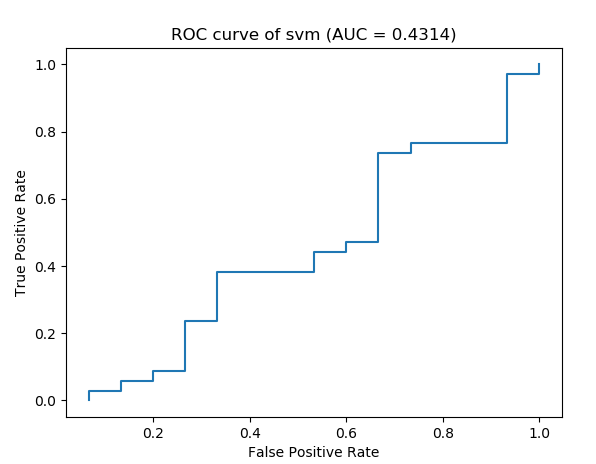• case2: 取全部数据，txt文件名为：evaluate_result
'''

'''
#绘制二分类ROC曲线
import matplotlib.pyplot as plt
from math import log, exp, sqrt

evaluate_result = './evaluate_result.txt'

db  = [ ]   #[score,nonclk,clk]
pos, neg = 0, 0
with open(evaluate_result, 'r') as fs:
for line in fs:
nonclk, clk, score = line.strip().split('\t')  #变成列表list形式
nonclk = int(nonclk)
clk = int(clk)
db.append([score,nonclk,clk])

score = float(score)
neg = neg + nonclk
#print("neg数量====", neg, end = '')
pos = pos + clk
#print(",  pos数量====", pos)

#print("db= ",db)

db = sorted(db, key=lambda x:x, reverse=True)  #对ad的score进行降序排序
#print("db_reverse= ",db)

#计算ROC坐标点
xy_arr = [ ]
tp, fp = 0., 0.

for i in range(len(db)):
fp += db[i]
#print("fp ===", fp, end = '')
tp += db[i]
#print(",   tp ===",tp)
xy_arr.append([fp/neg, tp/pos])   #fp除以negative数目， tp除以positive数目。作为坐标点
#print(xy_arr)  #[[0.06666666666666667, 0.0], [0.06666666666666667, 0.029411764705882353],...]

# 计算曲线下面积
auc = 0.
prev_x = 0
for x, y in xy_arr:   #  x= fp/neg， y = tp/pos
if x != prev_x:
auc += (x - prev_x) * y  #矩形面积累加
prev_x = x

print("The auc is %s" %auc)

x = [ v for v in xy_arr]
y = [ v for v in xy_arr]
plt.title("ROC curve of %s (AUC = %.4f)" % ('svm',auc))
plt.xlabel("False Positive Rate")
plt.ylabel("True Positive Rate")
plt.plot(x, y)
plt.show()


The auc is 0.5788039199235478### 参考资料：

posted on 2018-09-09 14:56  星辰之衍  阅读(1677)  评论(0编辑  收藏